# What's behind matrix multiplication?Matrix multiplication is not easy to understand.

Even looking at the definition used to make me sweat, let alone trying to comprehend the pattern. Yet, there is a stunningly simple explanation behind it.

Let's pull back the curtain!

First, the raw definition. This is how the product of $A$ and $B$ is given. Not the easiest (or most pleasant) to look at.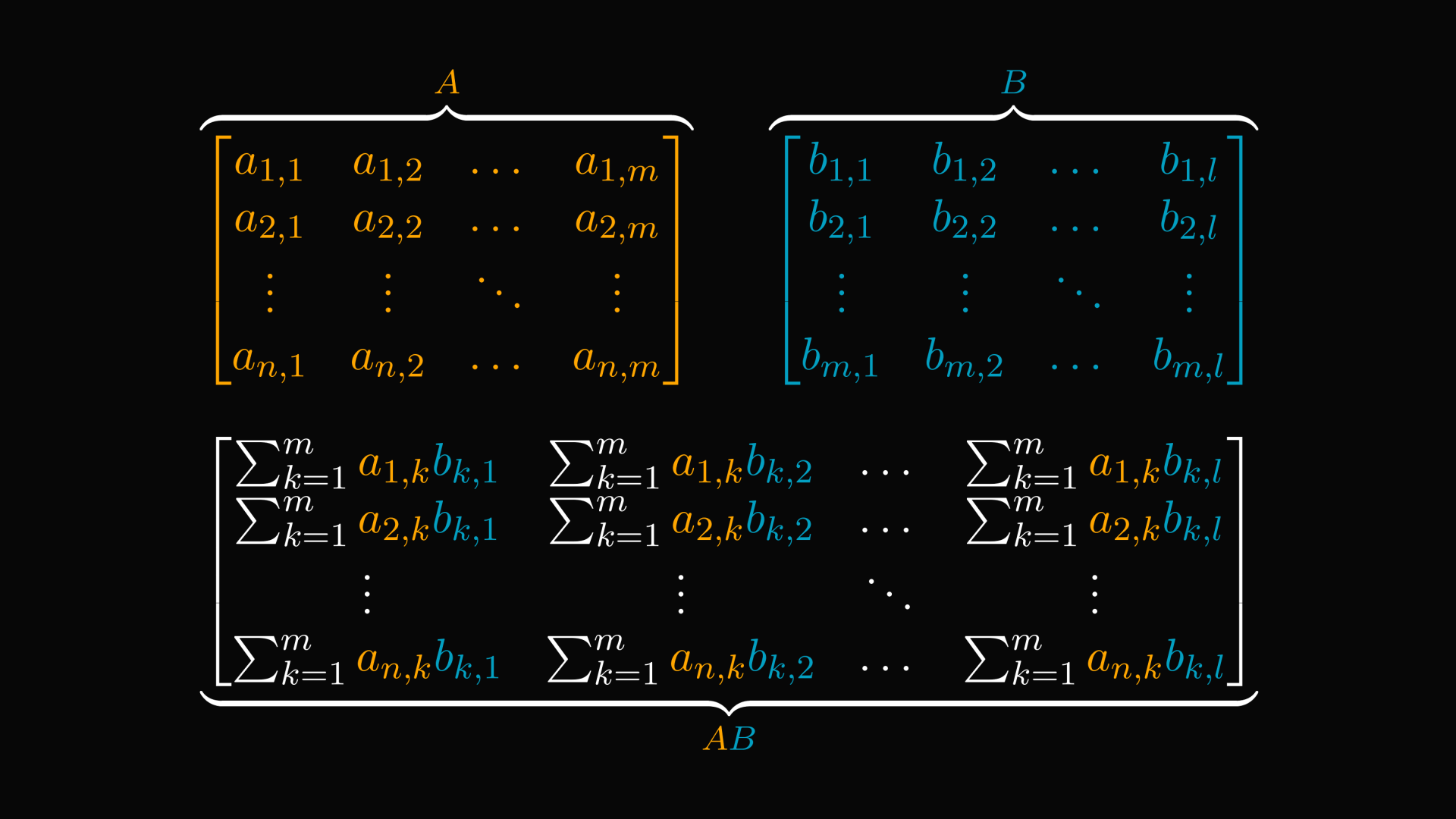We are going to unwrap this. Here is a quick visualization before the technical details. The element in the $i$-th row and $j$-th column of $AB$ is the dot product of $A$'s $i$-th row and $B$'s $j$-th column.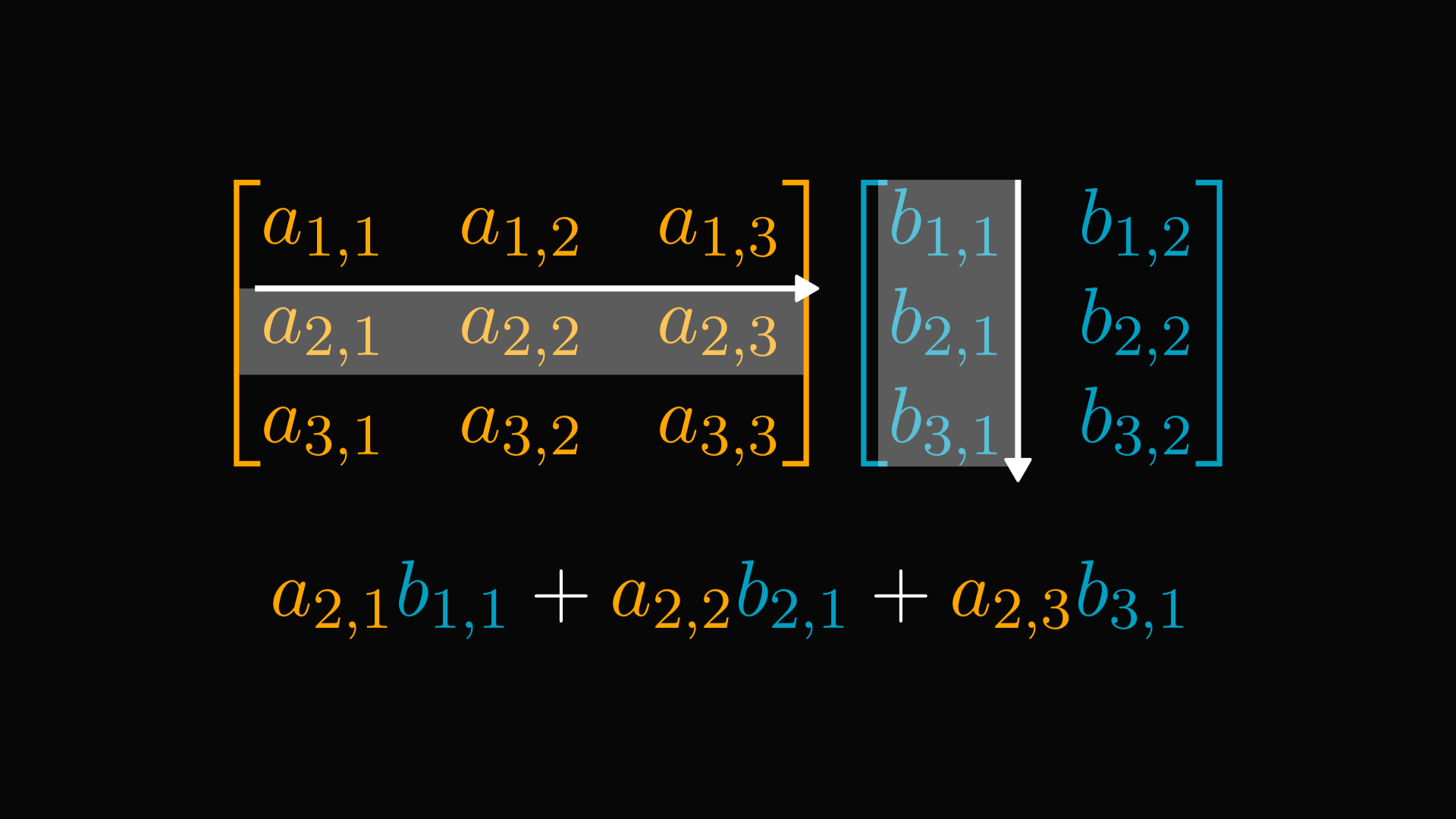Now, let's look at a special case: multiplying the matrix $A$ with a (column) vector whose first component is $1$, and the rest is $0$. Let's name this special vector $e_1$. Turns out that the product of $A$ and $e_1$ is the first column of $A$.Similarly, multiplying $A$ with a (column) vector whose second component is $1$ and the rest is $0$ yields the second column of $A$.

That's a pattern!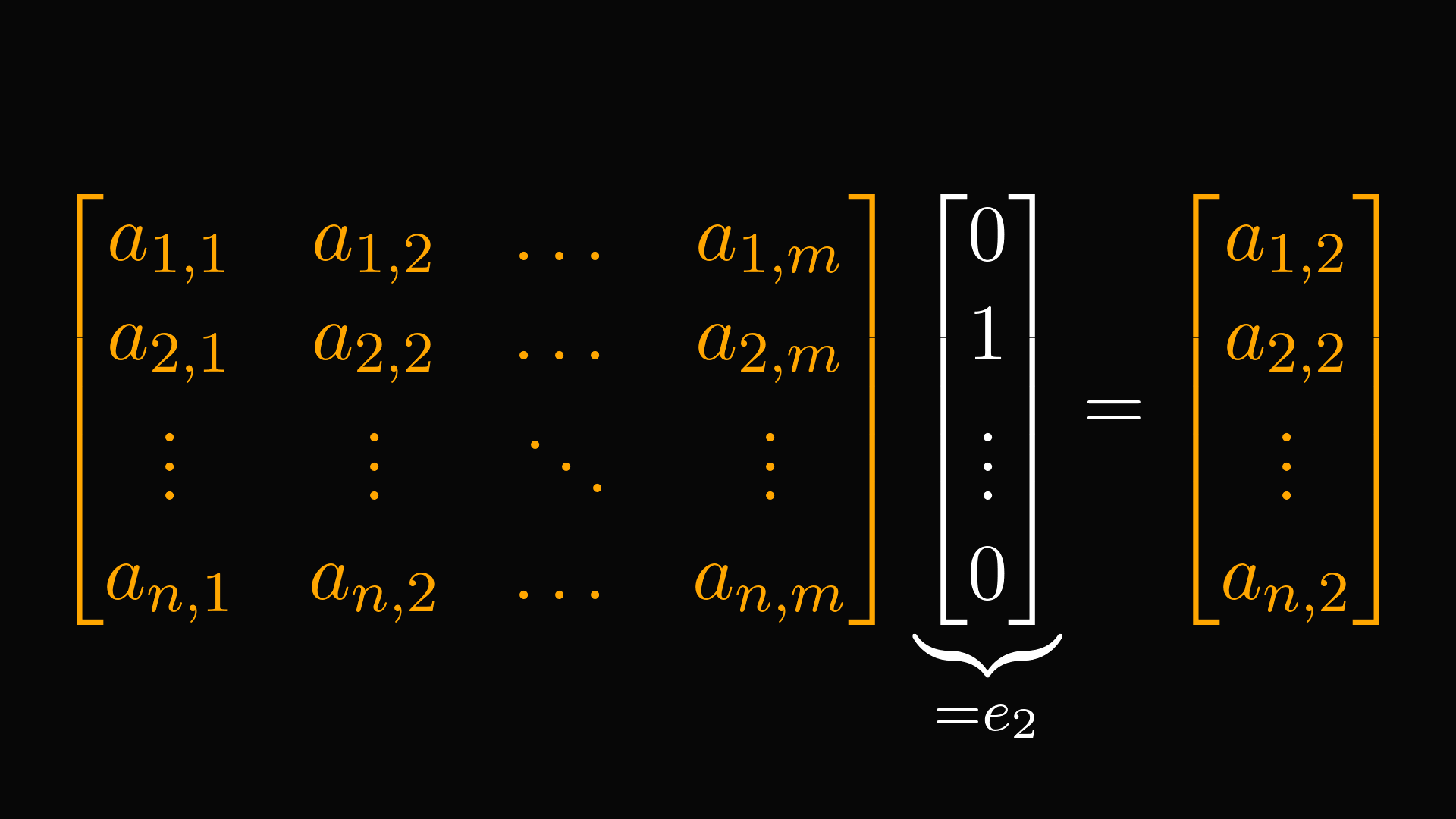By the same logic, we conclude that $A$ times $e_k$ equals the $k$-th column of $A$.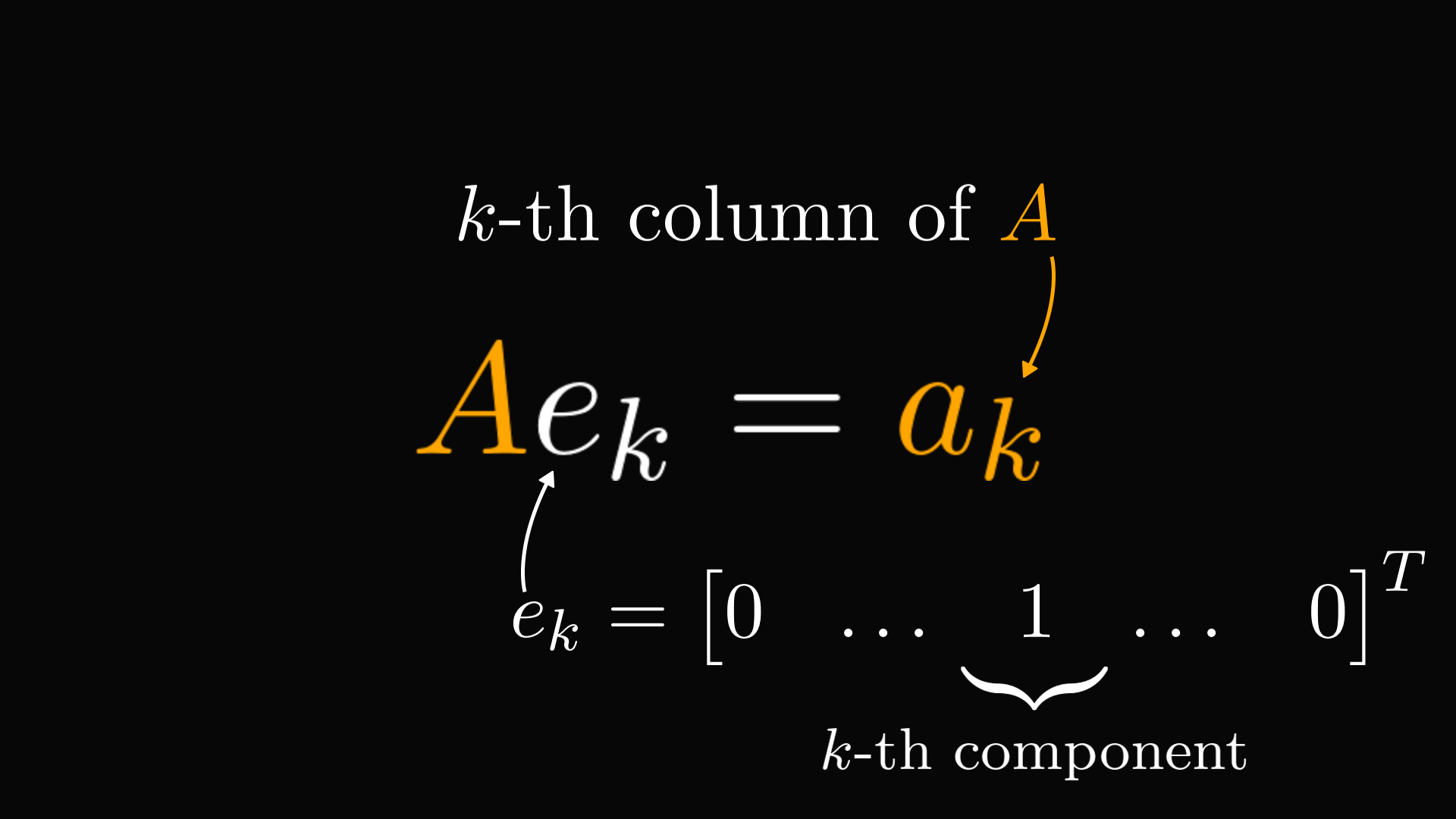This sounds a bit algebra-y, so let's see this idea in geometric terms. Yes, you heard right: geometric terms.

Matrices represent linear transformations. You know, those that stretch, skew, rotate, flip, or otherwise linearly distort the space. The images of basis vectors form the columns of the matrix.

We can visualize this in two dimensions.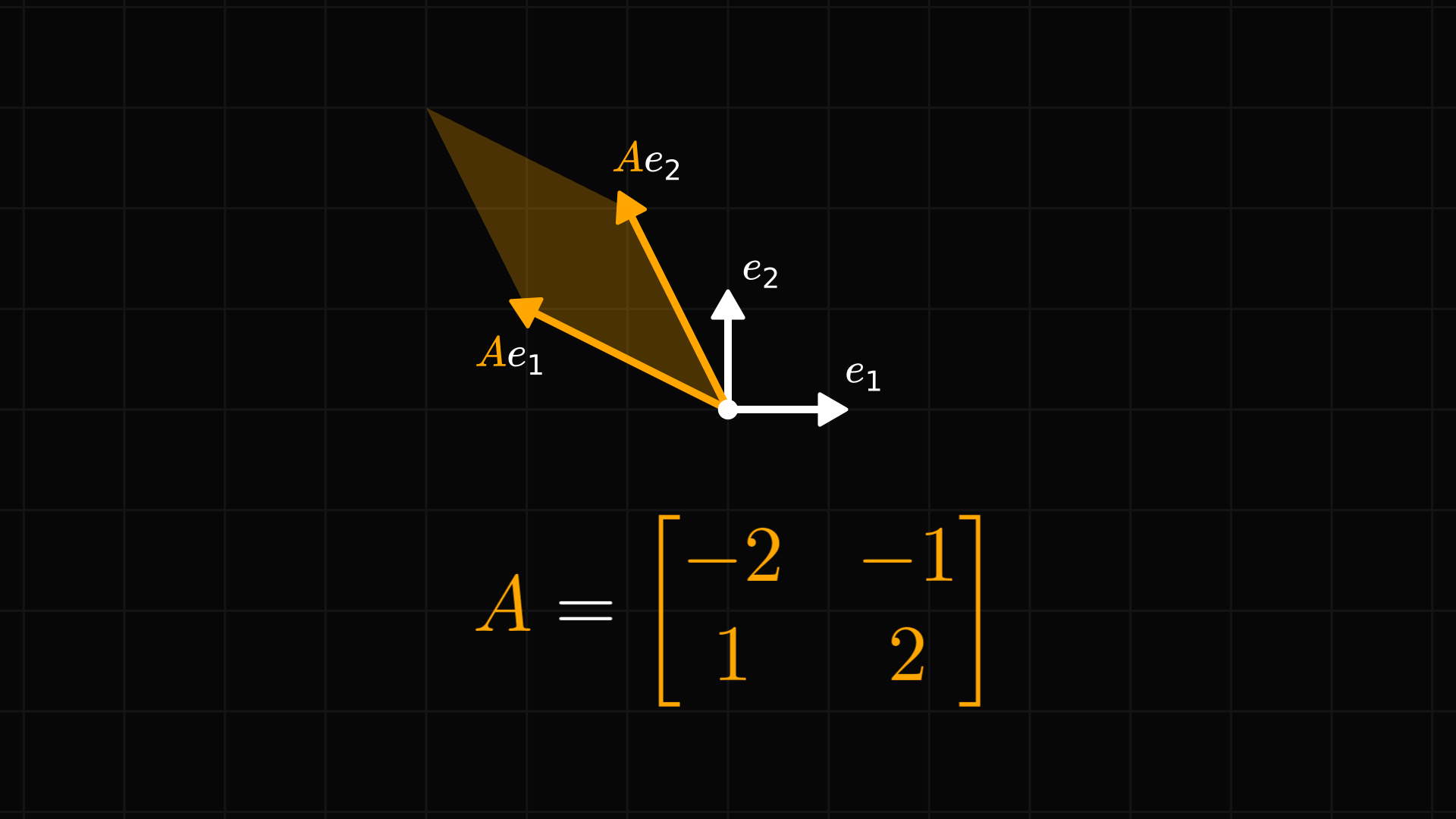Moreover, we can look at a matrix-vector product as a linear combination of the column vectors. Make a mental note of this, because it is important.(If unwrapping the matrix-vector product seems too complex, I got you. The computation below is the same as in the above, only in vectorized form.)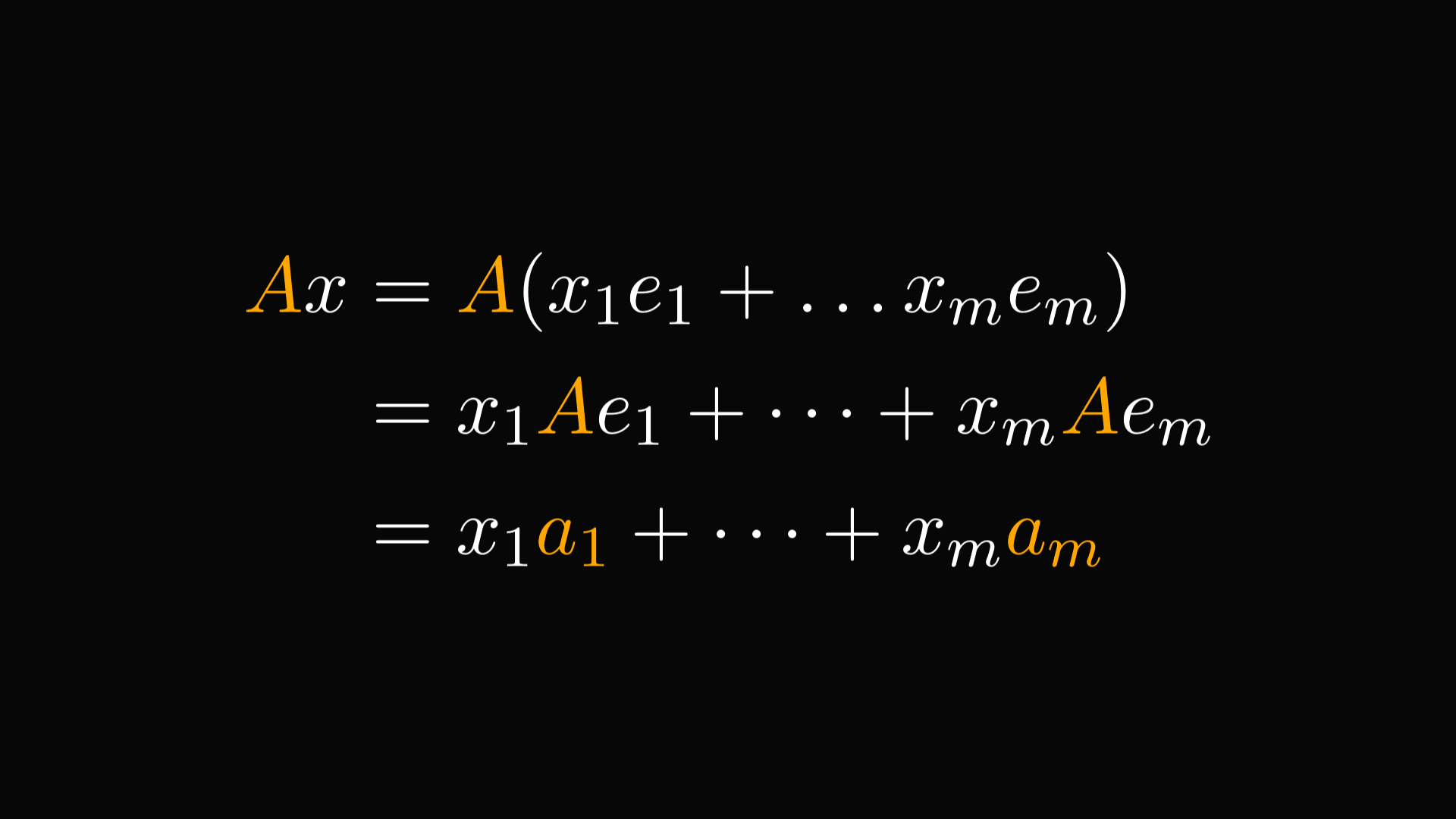Now, about the matrix product formula. From a geometric perspective, the product $AB$ is the same as first applying $B$, then $A$ to our underlying space.Recall that matrix-vector products are linear combinations of column vectors. With this in mind, we see that the first column of $AB$ is the linear combination of $A$'s columns. (With coefficients from the first column of $B$.)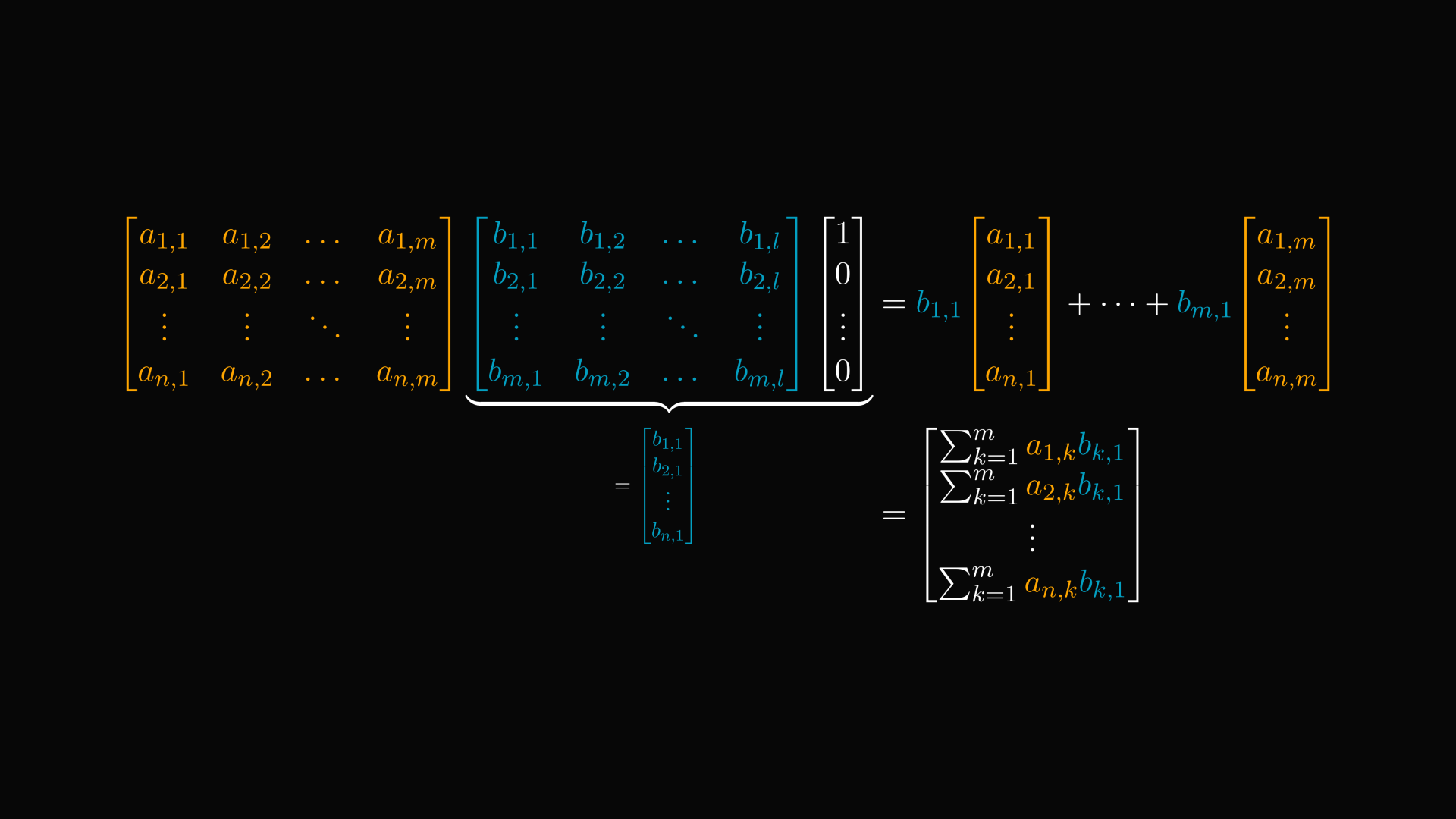We can collapse the linear combination into a single vector, resulting in a formula for the first column of $AB$. This is straight from the mysterious matrix product formula.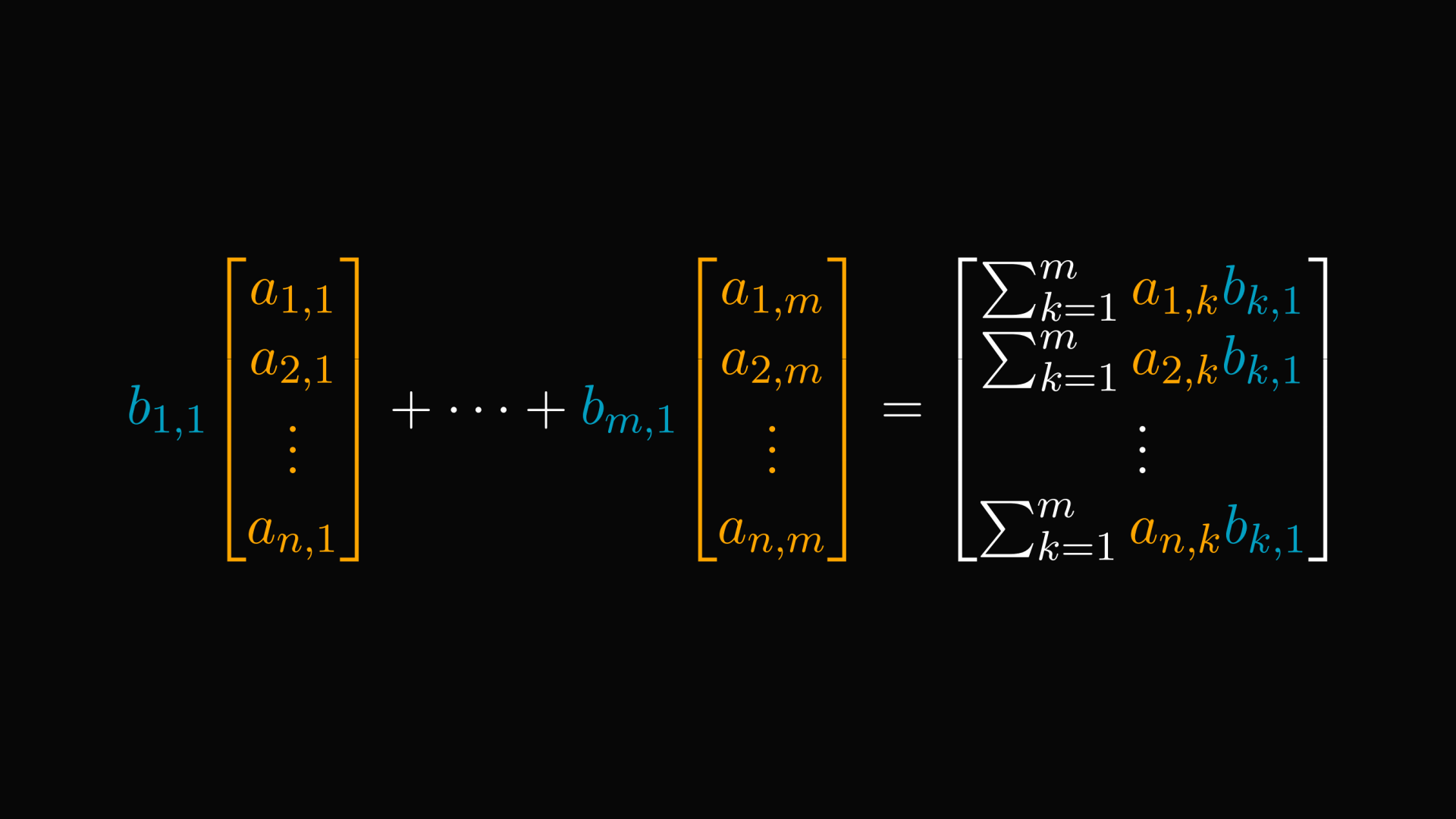The same logic can be applied, thus giving an explicit formula to calculate the elements of a matrix product.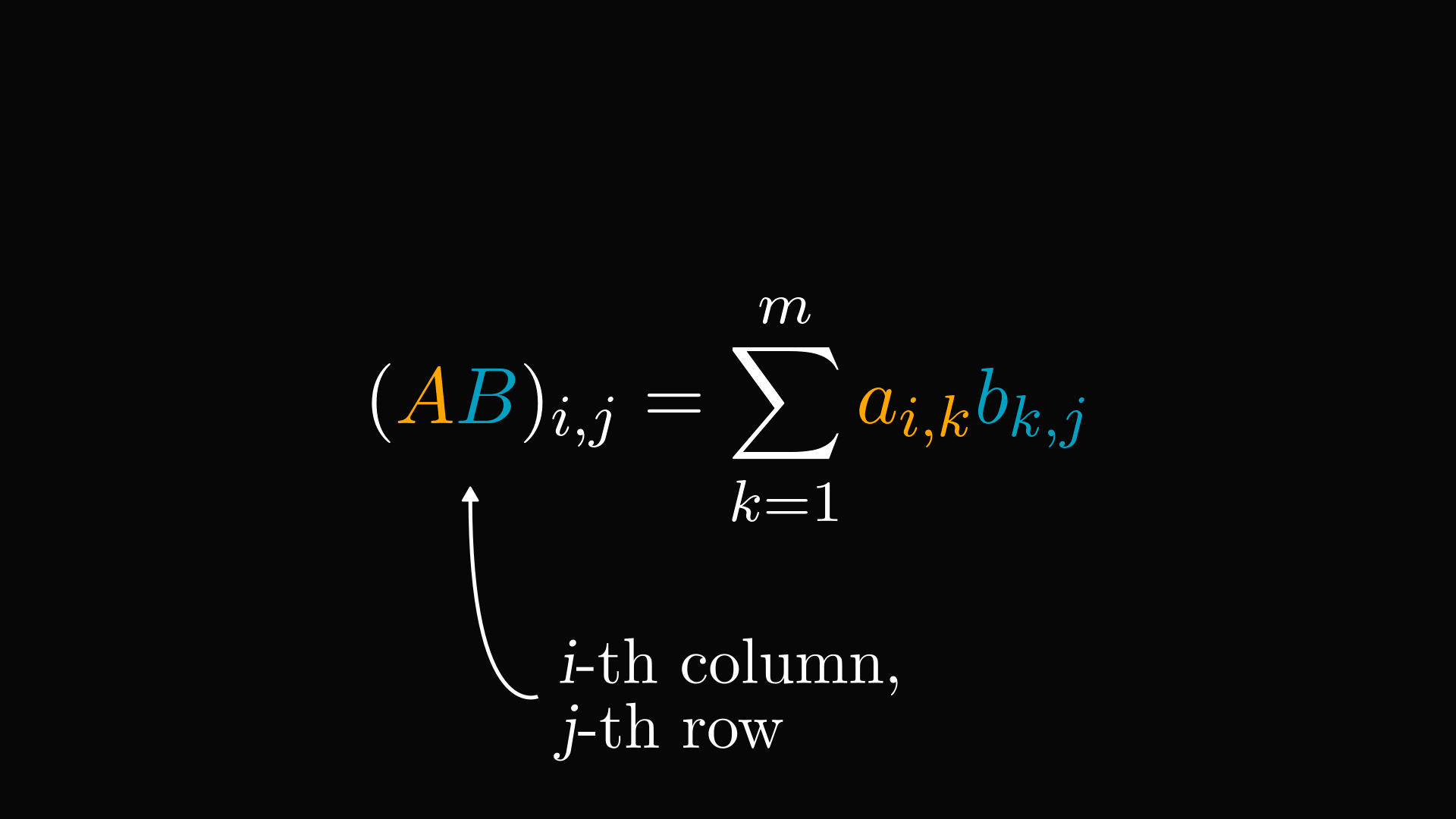Linear algebra is powerful exactly because it abstracts away the complexity of manipulating data structures like vectors and matrices. Instead of explicitly dealing with arrays and convoluted sums, we can use simple expressions $AB$.

That's a huge deal.

Peter Lax sums it up perfectly: "So what is gained by abstraction? First of all, the freedom to use a single symbol for an array; this way we can think of vectors as basic building blocks, unencumbered by components."